The experimental neutrino physics group at CENPA is involved in a number of different experiments to both understand the nature of the neutrino and to use neutrinos as a probe to understand the earth, sun, and supernovae. Over the past decade we have played an important role in determining that neutrinos change flavor as they propagate, and therefore have mass. These measurements have placed both an upper bound and lower bound on the neutrino mass. We are now involved in a number of experiments that could measure the absolute neutrino mass. Some of these experiments rely on so-called neutrinoless double beta decay, which if observed would mean that neutrinos are their own antiparticle and lepton number is not conserved. Due to their limited interactions with matter, neutrinos are an ideal probe to understand the energy production mechanism with the earth, sun, and core-collapse supernovae.## Neutrino ResearchSNO

The SNO experiment completed data taking in 2006, and will complete the final analysis this year. We measured that the total flux of neutrinos from the sun matched theoretical predictions, and that apparent deficit in previous experiments was due to neutrino oscillations. By measuring the flux of electron neutrinos from the sun, we also precisely determined the neutrino oscillation parameters.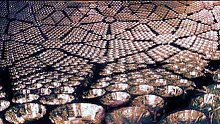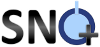SNO+

The SNO+ experiment will perform an essentially background-free measurement of neutrinoless double-beta decay (0νββ) in approximately 200 kg of 150Nd with the goal of observing lepton number violation and possibly determining the neutrino mass. This experiment could also measure neutrinos from both the sun and earth, which would test theories of energy production in both. We are currently involved in developing the data acquisition system and simulations.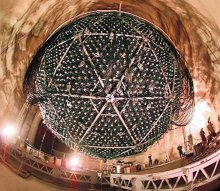KATRIN

The KATRIN experiment is designed to measure the mass of the electron neutrino directly with a sensitivity of 0.2 eV. It is a next generation tritium beta-decay experiment scaling up the size and precision of previous experiments by an order of magnitude as well as the intensity of the tritium beta source. We are currently developing the electron detector for this experiment.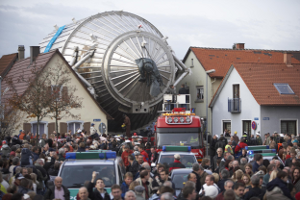The MAJORANA Neutrinoless Double-Beta Decay Experiment

The MAJORANA Experiment will perform an essentially background-free measurement of neutrinoless double-beta decay (0νββ) in 120 kg of 76Ge with the goal of determining the neutrino mass.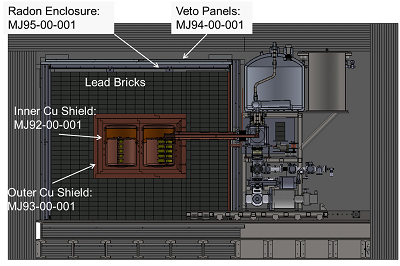Project 8

Project 8 is being developed as a corollary to the KATRIN neutrino mass search. By measuring the energy of electrons emitted in a tritium beta decay we can then infer the mass of the neutrino from the missing energy.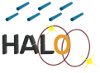HALO

The HALO experiment is designed to detect galactic core-collapse supernovas using a Pb-based neutrino detector. Such supernovae are expected to occur approximately three times per century with the first and last detection of a nearby supernova occurring in 1987. The next observation with new and larger detectors will provide a wealth of data for both particle physicists and astrophysicists. (link: http://www.snolab.ca/halo/index.html)